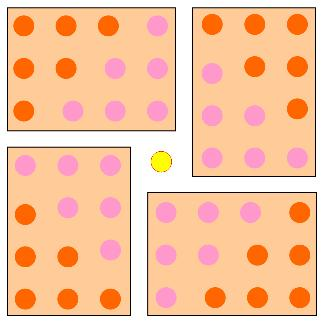You may also likeOdd Differences

The diagram illustrates the formula: 1 + 3 + 5 + ... + (2n - 1) = nÂ² Use the diagram to show that any odd number is the difference of two squares.Triangular Triples

Show that 8778, 10296 and 13530 are three triangular numbers and that they form a Pythagorean triple.Triangles Within Squares

Age 14 to 16Challenge Level

Well explained by Tom, from Cottenham Village College

The answer I got for $T_n$ is: $8T_n+1=(2n+1)^2$

The $2n+1$ part is because the diagram looks like this for $T_3$$2T_n$ form a rectangle $n$ by $n+1$

The four rectangles rotate around the centre and together make a square of side $n+(n+1)$

So we get the equation $8T_n+1=(2n+1)^2$